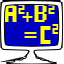# Mathomatic

## Do symbolic mathematics and quick calculations

Respects Freedom

Mathomatic implements most of the rules of algebra for the mathematical operators +, -, *, /, % (modulus), and power (including roots).

Mathomatic can symbolically:

* combine and solve equations, * completely simplify and compare expressions and equations, * do simple calculus transformations and series, * do standard, complex number, modular, and polynomial arithmetic, * generate optimized C, Java, and Python language code from simplified equations, * plot expressions with gnuplot in two or three dimensions, * do quick calculations, colorize expressions, etc.

### Information

Unknown

Versions

16.0.5-4 (landing)

16.0.5-4 (byzantium)

16.0.5-1 (amber)

Category

Education